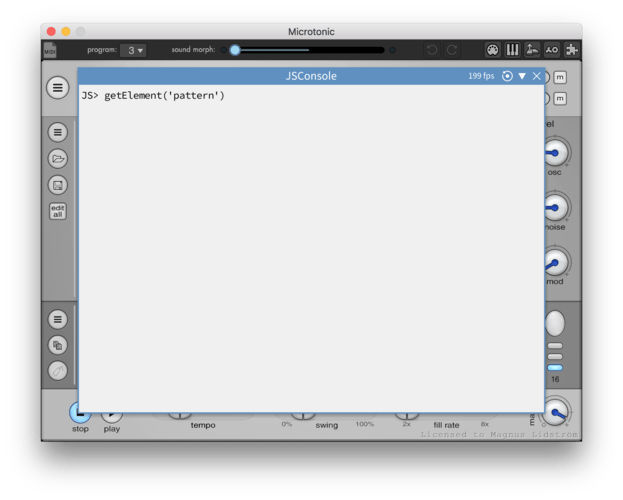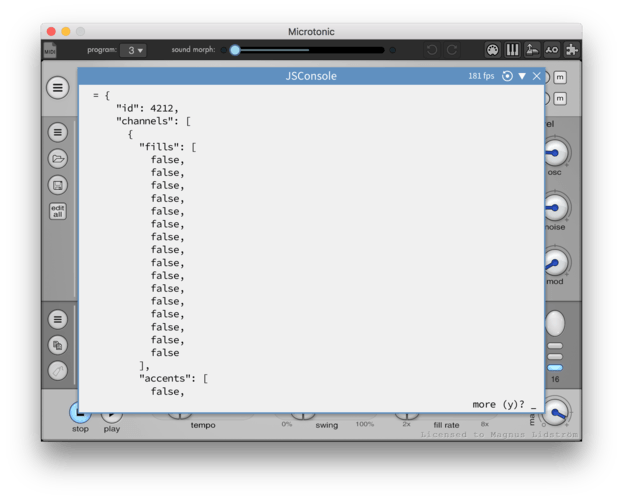JBDS192 views6 posts
• #### JBDS1 2023-03-19T00:05:56Z

include('stdlib.pika');
include('resources/mdk.pika');

for (ch = 1; ch <= CHANNEL_COUNT; ++ch) {
rmsp = function() {
args(@patch, @ch);
var selectedPattern = selected('pattern');
var numSteps = selectedPattern.steps;

for (var i = 0; i < numSteps; i++) {
selectedPattern.setStepData(i, {
'@Note': random(0, 127),
'@Velocity': random(0, 127)
});
};
};

stamp(rmsp);
}

Error: Script error: Expected '{'.

include('stdlib.pika');
include('resources/mdk.pika');

function RandomPattern() {
var selectedPattern = selected('pattern');
var numSteps = selectedPattern.steps;

for (var i = 0; i < numSteps; i++) {
selectedPattern.setStepData(i, {
'Note': random(0, 127),
'Velocity': random(0, 127),
'Muted': random() < 0.5
});
}
}

error

include('stdlib.pika');
include('resources/mdk.pika');

function randomizePattern() {
var selectedPattern = selected('pattern');

// Get the number of steps in the pattern
var numSteps = selectedPattern.steps;

// Randomize notes and velocities for each step
for (var i = 0; i < numSteps; i++) {
selectedPattern.setStepData(i, {
'Note': random(0, 127),
'Velocity': random(0, 127),
});
}
}

var selectedPattern = selected('pattern');

// Get the number of steps in the pattern
var numSteps = selectedPattern.steps;

// Read notes and velocities for each step
for (var i = 0; i < numSteps; i++) {
var stepData = selectedPattern.getStepData(i);
println("Step " + i + ": Note = " + stepData.Note + ", Velocity = " + stepData.Velocity);
}
}
error

include('stdlib.pika');

function randomizePattern() {
var selectedPattern = selected('pattern');
var numSteps = selectedPattern.steps;

for (var i = 0; i < numSteps; i++) {
selectedPattern.setStepData(i, {
'Note': random(0, 127),
'Velocity': random(0, 127),
'Muted': random() < 0.5
});
}
}

randomizePattern();

error .. nothing works....how i can make this work???
i know we have this function but i m trying to create somethis simple so i can learn.Thank you

• #### Magnus Lidström 2023-04-03T14:31:08Z

Hi. Hehe, this looks like a strange mix between PikaScript and JavaScript. Microtonic still supports these two different script languages, but PikaScript support is for legacy only. We've moved away from that and use only JavaScript for our new scripts.

I assume you know the full documentation for scripts is available at https://github.com/malstrom72/microtonic-scripts-sdk

getStepData and setStepData are not part of the SDK

I strongly suggest you start by installing the JSConsole script found on github, if you haven't done so already.

Next, learn about `getElement` and `setElement`. For example, to read the currently selected pattern, you use `getElement('pattern')`. You can type this in the console:and see the result:As you can see you will obtain a JS object for the current pattern with arrays like "fills", "accents" etc.

So for example to randomize the triggers of all channels, here is what you could write:

``````var p = getElement('pattern');
for (var ch = 0; ch < CHANNEL_COUNT; ++ch) {
for (var i = 0; i < PATTERN_STEP_COUNT; ++i) {
p.channels[ch].triggers[i] = (Math.random() < 0.5);
}
}
setElement('pattern', p);
``````
• #### JBDS1 2023-04-03T20:09:00Z

Hi thank you for answering and thank you for helping me understand... I have installed the JS console and Microtonic SDK, but all the time I'm getting errors, obviously because I'm trying to execute pika script in JS console JavaScript ( and because I'm not that good with scripting either,, but we all start from somewhere ).. And if I make a script in JavaScript I have to save the file as JavaScript ( js ) and Microtonic will execute that script as he is doing the same for the pika script? Thank you... I am trying to learn because I have some good ideas that we can use as scripts.

• #### JBDS1 2023-04-03T21:56:07Z

- Magnus Lidström wrote:
Hi. Hehe, this looks like a strange mix between PikaScript and JavaScript. Microtonic still supports these two different script languages, but PikaScript support is for legacy only. We've moved away from that and use only JavaScript for our new scripts.
I assume you know the full documentation for scripts is available at https://github.com/malstrom72/microtonic-scripts-sdk
getStepData and setStepData are not part of the SDK
I strongly suggest you start by installing the JSConsole script found on github, if you haven't done so already.
Next, learn about `getElement` and `setElement`. For example, to read the currently selected pattern, you use `getElement('pattern')`. You can type this in the console:

and see the result:

As you can see you will obtain a JS object for the current pattern with arrays like "fills", "accents" etc.
So for example to randomize the triggers of all channels, here is what you could write:
```
var p = getElement('pattern');
for (var ch = 0; ch < CHANNEL_COUNT; ++ch) {
for (var i = 0; i < PATTERN_STEP_COUNT; ++i) {
p.channels[ch].triggers[i] = (Math.random() < 0.5);
}
}
setElement('pattern', p);
```

JS Console:
S> var p = getElement('pattern');
JS> for (var ch = 0; ch < CHANNEL_COUNT; ++ch) {
!!! SyntaxError: Expected '}'
JS> for (var i = 0; i < PATTERN_STEP_COUNT; ++i) {
!!! SyntaxError: Expected '}'
JS> p.channels[ch].triggers[i] = (Math.random() < 0.5);
!!! ReferenceError: ch is not defined
JS> }
!!! SyntaxError: Syntax error
JS> }
!!! SyntaxError: Syntax error
JS> setElement('pattern', p);

The Microtonic execut corect the random script , the console dose not... i think i have to learn more first.. :)

• #### Magnus Lidström 2023-04-05T14:20:20Z

Yeah, the console only executes one line at a time. If you remove all line-feeds from the code and paste as one long line, it will work.

• #### JBDS1 2023-04-05T22:11:12Z

thank you ...

You need to be to post a reply